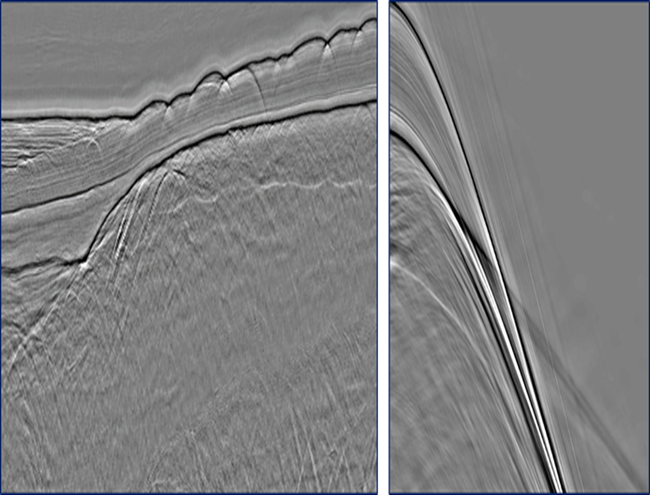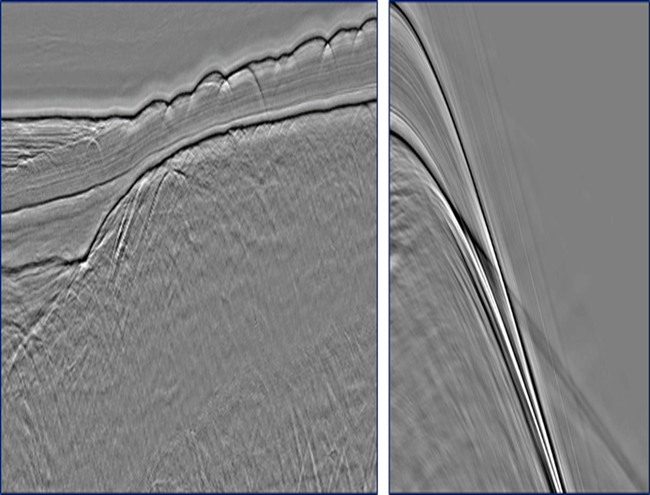# IME

## Interbed Multiple Elimination (IME)

IME utilizes a layer based, data driven algorithm to predict and model all internal multiples deriving from a given multiple generating horizon. The modeled multiples are passed into an adaptive subtraction algorithm for final subtraction from the data.

A variety of advanced adaptive subtraction algorithms are available, including least squares multidomain and curvelet subtraction, to subtract the predicted multiple model from the input dataset.

## Data example from East Coast Canada

Move the Slider Left or Right
to Visualize the ImagesSeismic section containing internal multiple energySeismic section following application of IME